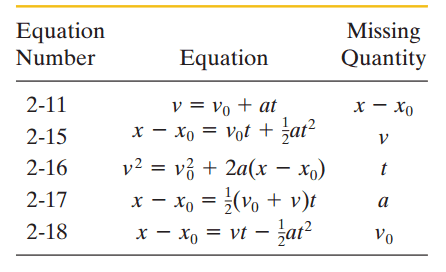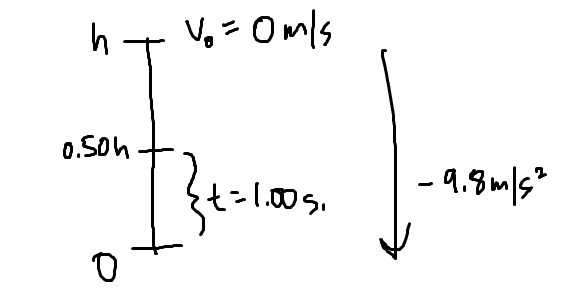# Beginner free-fall motion problem

## Homework Statement

A ball at rest is dropped from height 'h' and travels a distance of 0.50h in the final 1.00 seconds before it hits the ground.

Find the time and height of its fall.

## Homework Equations## The Attempt at a Solution

I drew a picture with the knowns:Obviously I first tried to solve for the total time, but couldn't because I didn't have total displacement of 'h'

So then I tried to solve for 0.50h - h, and got -0.50h = 0t - 1/2(9.8)(t^2) and still left with 2 unknowns.

Then I tried to solve for the bottom half, 0 - 0.50h but I am missing both initial and final velocity therefore there isn't any equation I can use to solve for the lower half since I will always end up with 2 unknown variables again.

I feel like I have exhausted my options because I don't see any other way to go about solving this, since there seems to be only two parts to this problem (the top part of the height and bottom part of the height.

Doc Al
Mentor
Write expressions for distance as a function of time for t1 & t2, which correspond to h/2 & h. How are those times related?

As we discussed previously, for a ball falling from rest, you can determine everything you want (in terms of the initial height). In particular, its velocity at 0.5h. This will then give you the complete set of initial conditions for the second part of the journey.

Write expressions for distance as a function of time for t1 & t2, which correspond to h/2 & h. How are those times related?

For this hint, I'd like to know how I should know, just by looking at this question, that I need to express the distances as a function for t1 & t2.

As we discussed previously, for a ball falling from rest, you can determine everything you want (in terms of the initial height). In particular, its velocity at 0.5h. This will then give you the complete set of initial conditions for the second part of the journey.

I tried to hold this mindset, but I still don't think I'm understanding what the determinism is for 1D kinematics.

For the velocity at 0.50h, I use equation 2-11 to find that v = -9.8t. But if I use equation 2-16, I get that v^2 = 9.8h. Are both of these supposed to be the same value?

Doc Al
Mentor
For this hint, I'd like to know how I should know, just by looking at this question, that I need to express the distances as a function for t1 & t2.
Well, you are given time and asked to find the distance. So what else would you do? (And I'm talking about the very kinematic formula that you tried using.)

For the velocity at 0.50h, I use equation 2-11 to find that v = -9.8t. But if I use equation 2-16, I get that v^2 = 9.8h. Are both of these supposed to be the same value?

Yes. You got the velocity in terms of h. Plug one into another, and you get time in terms of h (if you need it). With this velocity you could express everything in the second segment also in terms of h. Including the time spent in the second segment, which is given to you as a number.

Well, you are given time and asked to find the distance. So what else would you do? (And I'm talking about the very kinematic formula that you tried using.)

I thought about trying to solve for missing velocity for both of them so that I might be able to plug that into the equations to solve for time. It's not immediately clear to me that I need distances as functions for t1 and t2 first. Not sure why this is physically.

But for the top part, I get -0.50h = -(1/2)(9.8)(t_1)^2
And for the bottom, I get -0.50h = sqrt(9.8h) - (1/2)(9.8)(t_2)^2

I just replaced V_0 of the bottom part with the V_final of the top part since they are the same

Oh, I guess t_2 = 1.00s as the given...didn't see that until it was pointed out

Doc Al
Mentor
You'll have a much easier time if you don't try to directly write an expression for the bottom half. Instead, just write expressions for the first half (to h/2) and the full distance (h).

Yes. You got the velocity in terms of h. Plug one into another, and you get time in terms of h (if you need it). With this velocity you could express everything in the second segment also in terms of h. Including the time spent in the second segment, which is given to you as a number.

I'm not sure what this all means...I have the final velocity of the top part as t and height of the top part...how would any of those help me with the bottom part? For example if I plug in v = -9.8t_1 into v^2 = 9.8h, I get (-9.8t_1)^2 = 9.8h so in terms of h, it's 96.04(t_1)^2 / 9.8 which is just 9.8(t_1)^2 = h.

If I plug it into some equation for the bottom half, such as 2-15, I get:

0 - 0.50(9.8(t_1)^2) = v_0*1 - (1/2)(9.8)(1)^2 and for v_0 I can replace it with v = -9.8(t_1):

0.50(9.8(t_1)^2) = -9.8(t_1) - (1/2)(9.8) =
4.9(t_1)^2 = -9.8(t_1) - 4.9 =
0 = -4.9(t_1)^2 - 9.8(t_1) - 4.9

Which has just '-1' as a solution which isn't a valid time.

I thought about trying to solve for missing velocity for both of them so that I might be able to plug that into the equations to solve for time. It's not immediately clear to me that I need distances as functions for t1 and t2 first. Not sure why this is physically.

But for the top part, I get -0.50h = -(1/2)(9.8)(t_1)^2
And for the bottom, I get -0.50h = sqrt(9.8h) - (1/2)(9.8)(t_2)^2

I just replaced V_0 of the bottom part with the V_final of the top part since they are the same

Oh, I guess t_2 = 1.00s as the given...didn't see that until it was pointed out

For this one, the bottom part turned out to be a quadratic 0 = h^2 - 14.7h + 24.01 which has two positive solutions of 1.87 and 12.8. Both of these heights could be valid, I guess?

You'll have a much easier time if you don't try to directly write an expression for the bottom half. Instead, just write expressions for the first half (to h/2) and the full distance (h).

For the ball falling from h to h/2, I get (using equation 2-15): -h/2 = -(1/2)(9.8)(t_1)^2
And for h to 0 (the ground): -h = -(1/2)(9.8)(t_1 + 1)^2

Is this in the right direction? I don't know how to tell if I'm going the right way or not because I'm not sure what all these equations mean.

I'm not sure what this all means...I have the final velocity of the top part as t and height of the top part...how would any of those help me with the bottom part?

The final velocity in the top part is the initial velocity in the bottom part. So you could use 2-15: ## 0 - 0.5h = -\sqrt{gh}t - \frac {gt^2} 2 ##

Which should be the same equation you got with the other approach. Solve it for h.

The final velocity in the top part is the initial velocity in the bottom part. So you could use 2-15: ## 0 - 0.5h = -\sqrt{gh}t - \frac {gt^2} 2 ##

Which should be the same equation you got with the other approach. Solve it for h.

Sorry, where did the negative sign in front of the V_0 come from?

Sorry, where did the negative sign in front of the V_0 come from?

It is not in front of ##v_0##. It is the value of ##v_0## that is negative. The ball is falling down.

Doc Al
Mentor
For the ball falling from h to h/2, I get (using equation 2-15): -h/2 = -(1/2)(9.8)(t_1)^2
And for h to 0 (the ground): -h = -(1/2)(9.8)(t_1 + 1)^2

Is this in the right direction? I don't know how to tell if I'm going the right way or not because I'm not sure what all these equations mean.
Exactly the right direction. Those equations relate time to distance, which is what you want.

Now you have two equations and two unknowns. Solve!

Exactly the right direction. Those equations relate time to distance, which is what you want.

Now you have two equations and two unknowns. Solve!

How come we couldn't relate time to velocity? Is it because the question asked for time and distance? If so, why couldn't we relate distance to time instead?

Doc Al
Mentor
How come we couldn't relate time to velocity?
You could, but that just adds a step. You'll have to figure out the velocity. But there are usually several ways to attack a problem. (Just jump in!)
Is it because the question asked for time and distance? If so, why couldn't we relate distance to time instead?You are relating distance to time.

You could, but that just adds a step. You'll have to figure out the velocity. But there are usually several ways to attack a problem. (Just jump in!)You are relating distance to time.

Oh, I thought there was a difference in relating time to distance and distance to time.

Knowns are v0=0 and t2=1sec and a=g.

3 unknowns are v1, t1 and h.
So you need 3 equations to solve the problem.

v1=at1 .........(1)
0.5h=0.5a(t1)2......(2) 1st. segment of the drop initial velocity=0.
0.5h=v1+0.5a..............(3) 2nd segment with t2=1,initial velocity=v1